# Derivative vs Integral (3 Key Things To Know & Comparison)

In calculus, derivatives and integrals are two different concepts – but they are related in an important way.  Both tell us something about a function and its behavior, but it helps to know exactly how they are used.

So, what is the difference between a derivative and an integral?  The derivative f’(x) of a function f(x) gives the slope of the tangent line at a point. The integral f(x) of a function f’(x) gives the area between f’(x) & the x-axis on the interval [a, b]. The Fundamental Theorem of Calculus connects these two concepts with the equation ∫abf’(x)dx = f(b) – f(a).

To put it simply, derivatives and indefinite integrals (antiderivatives) act like inverses (opposites).  That is, if we take the integral of a function and then take the derivative of the integral, we get the same function back.

In this article, we will talk about derivatives, integrals, and the difference between the two concepts.  We’ll also look at some examples to show how the ideas are connected.

Let’s get started.

Hey! If you need to learn more about derivatives, integrals, and other math concepts for physics, check out this course:

Advanced Math For Physics: A Complete Self-Study Course

## Derivative vs Integral

A derivative tells us about the slope of a curve at a given point, while an integral tells us about area under a curve on a given interval.  More specifically:

• The derivative of a function tells us the slope of a tangent line the function at a given point.
• The integral of a function tells us the area between the function and the x-axis on an interval.

The table below compares derivatives and integrals side-by-side.

Before we dive in deeper to connect derivatives and integrals, let’s define each one.

### What Is A Derivative?

The derivative of a function tells us how a function changes at a given point.  That is, a derivative tells us how fast a function is increasing or decreasing at a point (“slope” or “rate of change”).

For a function f(x), the derivative f’(x) is defined by:

• f’(x) = limh->0 [f(x+h) – f(x)] / h

This is called the limit definition of the derivative.

At a specific point x = a, the value f’(a) is the slope of the tangent line to f(x) at that point.  You can see the graph of a function and the tangent line at a given point below.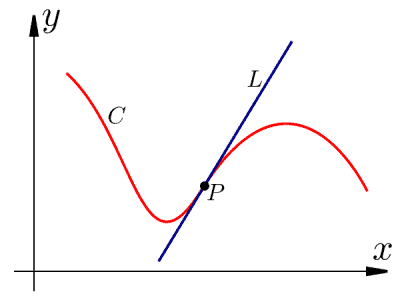This image shows the tangent line, L, to the function curve, C, at the point P. The slope of the line L is the derivative of the function, evaluated at the point P.

In other words, the tangent line for f(x) at x = a has the form y = mx + b with m = f’(a); that is, y = f’(a)x + b.

The best analogy to think about derivatives is a moving object, like a car or a plane:

• The function f(x) is the position of the car (where is the car at time x?)
• The derivative function f’(x) is the velocity of the (how fast is the car going at time x?)

We can extend this analogy to the second derivative f’’(x), which corresponds to the acceleration of the car (how fast is the velocity changing at time x?)

### What Is An Integral?

The integral of a function tells us the area under the curve on a given interval.  That is, an integral tells us the area between a function and the x-axis (this area is positive if above the x-axis, and negative if below the x-axis).

For a function f(x), the integral f(x) on the interval [a, b] is denoted by:

• abf(x)dx

On a specific interval [a, b], the value of the integral ∫abf(x)dx is the area between f(x) and the x-axis from x = a to x = b.  You can see the graph of a function and the area under the curve (integral) below.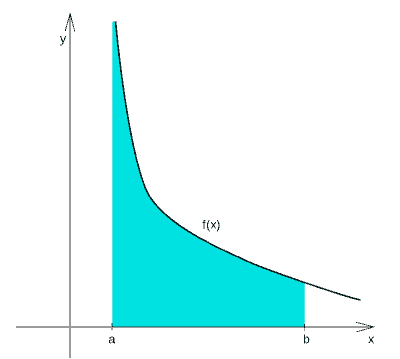The integral of f(x) on the interval [a, b] is the area under the curve f(x), or the area between f(x) and the x-axis.

The best analogy to think about integrals is a moving object, like a car or a plane:

• The function v(x) is the velocity (how fast is the car going at time x?)
• The function f(x) is the position of the car (where is the car at time x?)

If we take the integral of the velocity function on a time interval [a, b], we get the change in position over that time period.  In other words:

• Change in position from time a to time b =  ∫abv(x)dx

We can extend this analogy to the acceleration function a(x) which corresponds to the acceleration of the car (how fast is the velocity changing at time x?). We can also write:

• Change in velocity from time a to time b =  ∫aba(x)dx

Keep in mind that there are two types of integrals: definite integrals and indefinite integrals.

#### Definite Integral

A definite integral has arguments a and b (lower and upper bounds) indicated below and above the integral sign ∫.  For example, for the integral ∫abf(x)dx, we have:

• A lower bound of x = a
• An upper bound of x = b

This indicates that we want the area between f(x) and the x-axis from x = a to x = b, as shown below.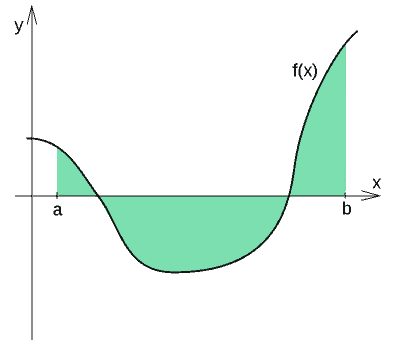The definite integral of the function f(x) with lower bound x = a and upper bound x = b gives the area between the curve f(x) and the x-axis. Any area above the x-axis is positive, and any area below the x-axis is negative.

A definite integral has a specific value, based on the arguments (upper and lower bounds), or we can find a specific function based on initial conditions (an initial value problem).

#### Indefinite Integral

An indefinite integral has no arguments (no upper and lower bound).  Instead, we take a general antiderivative, which will include an unknown constant term C.

So, the indefinite integral of the function f’(x) is f(x) + C, where C is a constant.  As mentioned above, we can find the value of C if we are given specific values of x and y for the function.

The notation for the indefinite integral of f(x) is ∫f(x)dx.

### Fundamental Theorem Of Calculus

The Fundamental Theorem of Calculus shows us the connection between the concepts of derivatives and integrals.

If f(x) is a real-valued function and continuous on the interval [a, b], then define F(x) by:

• F(x) = ∫axf(t)dt

Then the function F(x) is an antiderivative of f(x).  In other words, F’(x) = f(x).

Furthermore, F(x) is continuous on [a, b] and differentiable on (a, b).

A corollary, which we mentioned earlier, says that:

• abf(x)dx = F(b) – F(a)

In other words, the area under the curve f(x) on the interval [a, b] tells us how the function F(x) changes from a to b.

## Examples Of Derivatives & Integrals

It will be much easier to see how derivatives and integrals work (and how they are connected) with some examples.

### Example 1: Finding Velocity From Position

Let the function f(x) = 30x give the position of a car (in miles north of Boston) at time x >= 0.  Since f(x) is the position function, we can take the derivative to get velocity function, v(x) = 30.  That is, f’(x) = v(x).

This tells us that the car is traveling at a constant speed of 30 miles per hour. We can see the graph of v(x), the car’s velocity, below:

If we find the area under the velocity curve on a given time interval, we can find out how far the car has traveled during that time.

For example, from time x = 0 to time x = 2, the area is 2*30 = 60 miles.  This makes sense, since the car traveled at 30 miles per hour for 2 hours .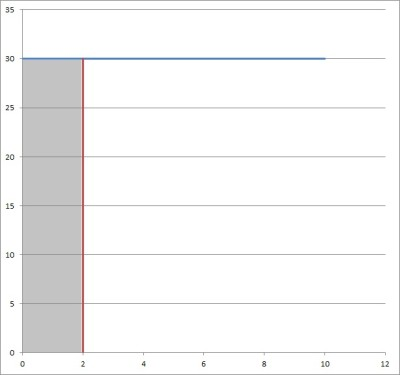The area under the velocity curve v(x) = 30 from x = 0 to x = 2 is 60 (2 hours times 30 miles per hour equals 60 miles).

Likewise, from time x = 0 to x = 5, the area is 5*30 = 150 miles, which you can see graphed below.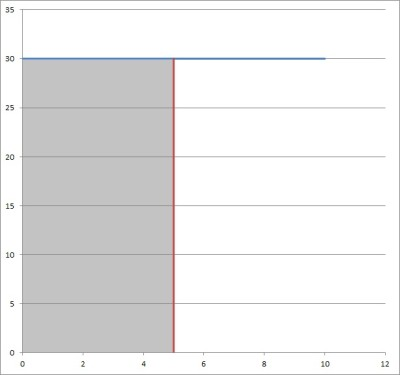The area under the velocity curve v(x) = 30 from x = 0 to x = 5 is 150 (5 hours times 30 miles per hour equals 150 miles).

The table below shows the cumulative area under the velocity curve from time x = 0.

If we graph these distances, we get the position function, shown below.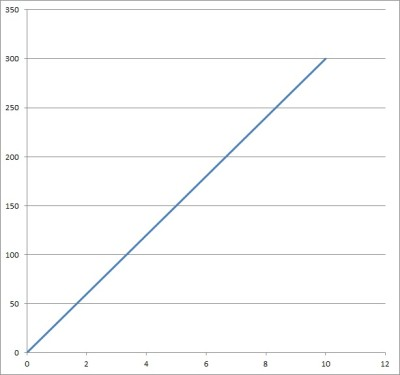This is the graph of the position function f(x) = 30x. Its derivative is the velocity function v(x) = 30.

Note: if the car had started 10 miles north of Boston, then the position function would be f(x) = 30x + 10, while the velocity function would still be v(x) = 30.

### Example 2: Finding Position From Velocity

Let the function g(x) = 200 – 10x be the velocity of a car in meters per second from time x = 0 to time x = 20.  The graph of the velocity function is shown below:

If we take the area under the velocity curve from time x = 0 to time x = b, we get the sum of the areas of two shapes:

• A rectangle with area bg(b) = b(200 – 10b) = 200b – 10b2
• A triangle with area b(200 – g(b))/2 = b(200 – (200 – 10b))/2 = b(10b)/2 = 5b2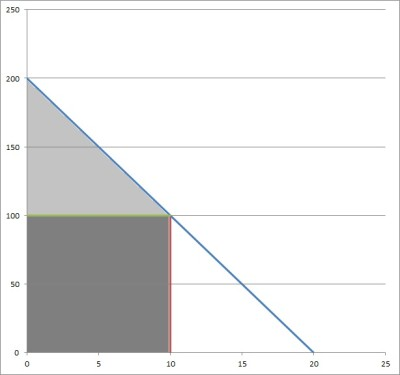The area under the curve has two parts: the rectangle (dark gray) and the triangle (light gray). Their sum is the integral of the velocity function g(x) from x = 0 to x = 10.

If we sum the areas of the individual shapes (rectangle and triangle) we get a total area of:

• Total Area = (200b – 10b2) + (5b2)
• Total Area = 200b – 5b2

The table below shows the value for the total area at times between x = 0 and x = 20.

If we graph these values, we get the position function G(x) for the car, which you can see below.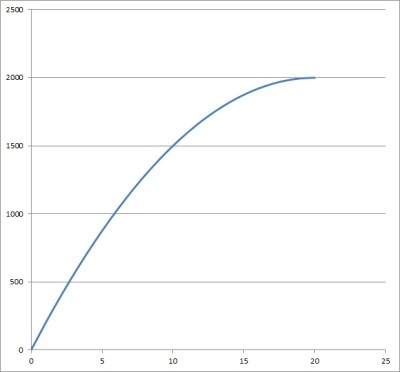This is the position function G(x) from x = 0 to x = 20. As time passes, the car slows down to a stop.

*Note: G’(x) = g(x), since velocity is the derivative of position.

## Conclusion

Now you know the difference between a derivative and an integral, as well as how the two concepts are related.

You can learn how to graph a function from its derivative here.

When taking derivatives, you might need to differentiate square roots – you can learn how to do it here.

You can learn about how to use derivatives and graphs to find function maximums here.